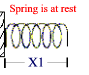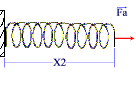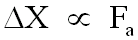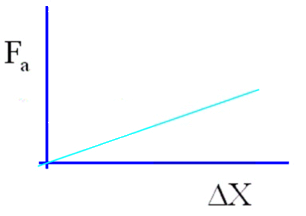#### Hooke's LawWhen a force (Fa) is applied to the spring, it will stretch by a certain amount.

Its new position will be X2The amount of stretch is given by the change from position X1 to position X2

This change in position is ∆X.

Where,

∆X.=  X2 - X1

The amount of stretch (∆X) is proportional to the applied force Fa.

i.e.Graphically, this relationship can be shown as:Hooke's Law  tells us that the force required to stretch a spring is given by

Fnet  =  k∆X

Therefore, the amount of "restoring force" required to bring the spring back to its equilibrium position is

Fnet  =  -k∆X